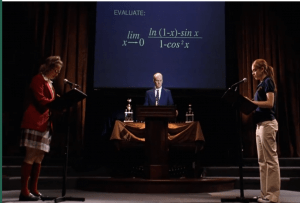# The limit does not exist!

Lindsay Lohan is really good at L’Hôpital’s rule.What’s more fetch than toaster strüdel, foot cream in a jar and a fertility vase from the Ndebele tribe? Arguably maths. More specifically, the mathematics of Mean Girls. If you haven’t seen Mean Girls, it’s a not-too-bad movie about teenage girls, teenage boys, teenage drama, and (a little bit of) maths.Stop trying to make fetch happen. It’s not going to happen.

It’s not exactly October 3rd*, but its been 13 years since Mean Girls came out, and

• $13 \times 2=26$
• $26+2\times2017=4060$
• $4060-3010=1050$, and
• $1050\log(2)+\frac{\pi}{1423211345}$ is… just a number.

What we’ll be looking at in this article is the last 10 minutes of the movie, where Cady and the Mathletes from North Shore compete in a maths state championship. After 87 minutes of play it’s a tie, and so the two teams enter the sudden death round, where the teams choose a member of the opposition (happening to both be female) to battle for the trophy.T. Pak as in ‘Trang Pak the grotsky little byotch’? No, just her brother.

In one corner, Cady Heron, AKA ‘Africa girl’, AKA the ‘used-to-be maths geek but not so much anymore after she met the Plastics but is kind of a geek again because her attempted sabotage of Regina George backfired and descended into chaos’. She is also the protagonist of the movie.

In the other, is Caroline Krafft, the female member of Marymount Prep, who “seriously needed to pluck her eyebrows, whose skirt looks like it was picked out by a blind Sunday school teacher, and had some 99-cent lipgloss on her snaggletooth”. This was when I realised, making fun of Caroline Krafft wasn’t going make me any better at writing this article (or maths for that matter).

The two were asked to solve the following;Caroline Krafft hastily states the incorrect answer, $-1$. If Cady manages to state the correct one then the North Shore mathletes ultimately win.

In the midst of all this Cady then has a miraculous flashback to the week that Aaron got his hair cut, and as she takes a moment to see straight pass Aaron’s face and onto the board behind, then has an epiphany (the kind we would like to have in our exams sometimes) and quickly realises the function diverges.Aaron Samuels is now a spin class instructor, so he won’t be expected to know what a factorial is anymore. Thank God.

So we don’t exactly know how Cady did this in under 10 seconds, but we can try to figure out the question for ourselves.

So the question reads$$\lim_{x\to 0} \frac{\ln(1-x)-\sin x} {1-\cos^2 x}.$$

We’ll consider L’Hôpital’s Rule, seeing as its the most straightforward way of going about it.

If we substitute $x$ with 0 we will find$$\frac{\ln(1)-\sin(0)} {1-\cos^2(0)} = \frac{0}{0}.$$
This satisfies the criteria for being able to apply L’Hôpital’s Rule, which is as follows,$$\lim_{x \to c} \frac{f(x)}{g(x)} = \lim_{x \to c} \frac{f'(x)}{g'(x)}.$$

Let’s apply it to our function, starting by considering the limit from the right;

\begin{align}
\lim_{x\to 0+} \frac{\frac{-1}{1-x}-\cos x} {\sin 2x} &= \lim_{x\to 0+} \frac{-x \cos x + \cos x + 1}{ (x-1) \sin 2x} \\
&= \lim_{x\to 0+} [-x \cos x + \cos x + 1] \lim_{x\to 0+}\left[\frac{1}{x-1}\right] \lim_{x\to 0+}\left[\frac{1}{\sin 2x}\right].
\end{align}
Now,\begin{align}
\lim_{x\to 0+} \left[-x\cos x +\cos x +1 \right] &=2, \\
\lim_{x\to 0+} \left[\frac{1}{\sin 2x } \right] &= \infty, \\
\lim_{x\to 0+} \left[\frac{1}{x-1} \right] &= -1,
\end{align}
so by properties of infinity we get
$$\lim_{x\to 0+} [-x \cos x + \cos x + 1] \lim_{x\to 0+}\left[\frac{1}{x-1}\right] \lim_{x\to 0+}\left[\frac{1}{\sin 2x}\right] = 2 \cdot \infty \cdot -1 = -\infty.$$

Repeating this method for the limit from the left, we will obtain the following,
$$\lim_{x\to 0-} \frac{-x \cos x + \cos x + 1}{ (x-1) \sin 2x} = +\infty.$$Just checking.

And so since the limit from the right and the left differ, the whole thing diverges. Yay.Another approach would be to look at the Taylor series approximations for the separate terms at $x$ near 0. We won’t do that though since it’s not as mathsy, but it might have been the quicker way to go, on the assumption that Cady really knows her Taylor series expansions. But“Cause the next time you see her she’ll be like, Ohh Kevin G!”